Volume 5, Issue 3, June 2016, Pages: 13-17

Analysis of the Influence of Dielectric Polarization on the Study of Electronic Orientation of Electromagnetic Field Propagating Through Oxide Based Thin Film

Emmanuel Ifeanyi Ugwu1, 2, 3, Lucas Williams Limbi4, Kalu Onyekachi2, Joshua Ezekiel Sambo2

1Department of Industrial Physics, Faculty of Science, Ebonyi State University, Abakalik, Nigeria

2Department of Physics, Faculty of Science, Federal University Lafia, Lafia, Nigeria

3Department Physic, Faculty of Natural Science, University of Jos, Jos, Nigeria

4Department of Physics, Faculty of Science, Nasarawa State University, Kefi, Nigeria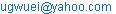(E. I. Ugwu)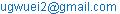(E. I. Ugwu)

Emmanuel Ifeanyi Ugwu, Lucas Williams Limbi, Kalu Onyekachi, Joshua Ezekiel Sambo. Analysis of the Influence of Dielectric Polarization on the Study of Electronic Orientation of Electromagnetic Field Propagating Through Oxide Based Thin Film. Advances in Materials. Vol. 5, No. 3, 2016, pp. 13-17. doi: 10.11648/j.am.20160503.11

Received: May 19, 2016; Accepted: June 7, 2016; Published: July 28, 2016

Abstract: In this work, we analyze the significance of dielectric polarizability on the study of electronic orientation in material which facilitates the understanding of the relative importance of the various contributions of the electronic polarizability of oxide based materials on electromagnetic wave propagation through it. In the mathematical formulation, Poisson equation in two dimensions was used to obtain polarizability constant, b. The polarizability constant was then varied and used in conjunction with the dielectric constants to assess the influence of b on wave propagation through the material. Based on this, electromagnetic wave equation was solved to obtain the wave function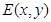in one and two dimensions with position and time for different in relation to various values of the polarizability constant. The graphs for real and complex values of the wave function in relation to polarizability were depicted respectively in figures. The graphs were found to display various characteristic behaviour for different polarizability constants.

Keywords: Dielectric Polarization, Polarizability Constant, Poison, Equation, Maxwell’s Equation, Electromagnetic Wave, Electronic Orientation, Wave Function, Analysis, Oxide Thin Film

Contents

1. Introduction

The polarizability is one of the quantitative measures of the dielectric polarization in study of electronic orientation in materials for which according to dielectric spectroscopic measurements emanate from the ionic [1,2] and molar polarization in the case of fluid and electronic in the case of solid and this is a quantity which is a measure of the polarizability of the individual molecule, whatever the state of aggregation of the materials. Ignoring gross features such as interface and structural irregularities, dielectric polarization can be electronic, ionic or orientation in nature The electronic and ionic polarizabilities arise from spring-like mechanism while the orientational polarizability does not.. In polar liquids, the molecules exhibiting such nature of polarizability have a permanent dipole moment μ invariant with variation of field for all realistic field magnitudes. The dielectric constant provides a common measure of solvent polarity that depict show much the inter-ionic Columbic interaction energy is reduced in a solvent compared to a vacuum.  Therefore, polarizable solvents, such as water and formamide, can effectively shield the charges on solute ions from one another, which is reflected in their high dielectric constants. The problem involved in ordering effect of the field energy and the disordering influence of thermal energy is such that each molecule with a permanent dipole moment μ makes a contribution to the polarization proportional to the field. . However, Langevin idea that said that the dipole must be aligned completely in order to achieve saturation value failed to consider dipole-dipole interaction  (i.e., he neglected Eμ) and hence the expression he obtained for polarization cannot be used without modification when such an interaction in magnetic field is significant especially as it is caused by induced dipoles, which gives rise to dispersions. At frequency at which dispersion caused by permanent dipoles occurs in those materials in which it exists, ionic polarization mechanisms are both fully operative, i.e. have low frequency or state value giving rise to completely real and complex components of polarization which maybe within ultra violet, visible and infra-red regions of the electromagnetic wave spectrum. From recent dielectric spectroscopy measurements it was shown that, as expected for an ionic medium, ion conductivity dominates the low frequency response and that a dielectric response was only measurable above about 1 GHz, but in most of the studied carried out already, it has benne found that exhibited rather modest dielectric constants within a range of 10 and 16 within 1GHz frequency domain, [6,7,8,9,10].

In this article, we present a simple model involving the analytical study of the electromagnetic field behaviour as it relates to influence of electronic orientation in dielectric polarization as it plays role in understanding the dielectric behavior of materials especially dielectric materials since induced electronic and ionic polarization gives rise to dispersion within the ultraviolet, visible, and infrared regions of E. M spectrum depending on the structure, composition and surroundings.

2. Theoretical Framework

In the theoretical frame work we considered the mathematical behaviour of a material placed within an externally applied field. Here we use Maxwell equation to link up the effect of polarizability on the propagating field which invariably culminated in the formulation of the poison equation which formed the main base for the analysis.

The orientational polarization for steady state as it relates to applied field, E is given by

Whereand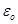stands for the orientational and electric polarizability of the material and permittivity of free space respectively.

The complex polarizability counterpart the correspondingly gives rise to complex polarization is given as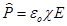(1)is complex susceptibility

This gives rise for an expression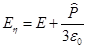(2)

Equations (2) and (3) can be solved to give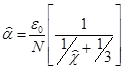(3)

We begin with Maxwell’s equations that relate electromagnetic field through material medium and the solid property of the material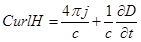(4)(5)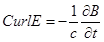(6)

where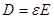and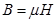ε and μ are the dielectric constant and permeability while j is the current due to the conduction electrons. The permeability is assumed to be unity.

Conversely, since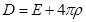,now is considered to result from charge density ρ/ given by

divρ= ρ/   (7)

Associated with j/ the current density that exists in parallel with the current density due to conduction electrons j given by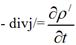(8)

From equations 4 and 5, we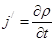(9)

Equation  represents the additional current [known as bound current] in conjunction to the current associated with the conduction electrons.

Conversely, we consider j to be absorbed into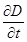, as we regard the entire solid as the medium through which electromagnetic wave propagates. For consistency, we take j=0 in equation [1 and 2] obtaining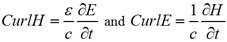(10)

For wave with time variation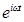travelling in the z-direction with E and H parallel to x-and y- directions respectively, we have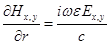(11)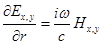(12)

On the account that field propagating through a metal induces polarization we have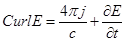(13)

of which with the time independent electric field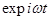, we can write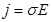(14)

Equations (13) and (15) enable us to write the expression interims of Poison equation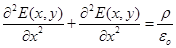(15)

which we now solve using method of separation of variable

Poisson equation in 2-dimension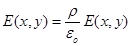(16)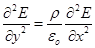(17)(18)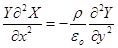(19)(20)

Let both equation be –b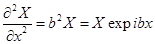(21)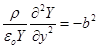(22)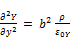(23)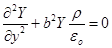(24)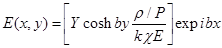(25)

Where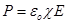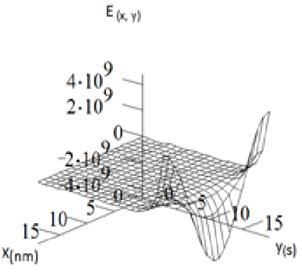Fig. 1. Wave function E(x,y) as a function of position and time for real part when b = 1.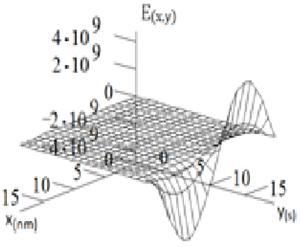Fig. 2. Wave function ψE(x, y) as a function of position and time when b = 1 for imaginary part.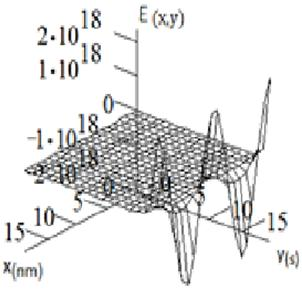Fig. 3. Wave function ψE(x,y) as a function of position and time for real part when b = 2.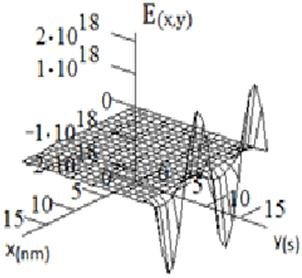Fig. 4. Wave function ψE(x,y) as a function of position and time when b = 2 for imaginary part for b =2.Fig. 5. Wave function E(x,y) as a function of position and time for real part when b = 5.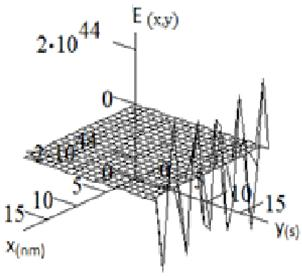Fig. 6. Wave function E(x,y) as a function of position and time when b = 5 for imaginary part for b=5.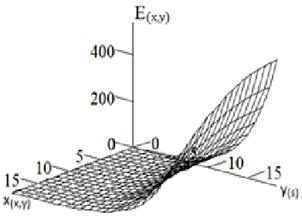Fig. 7. Wave function ψE(x,y) as a function of position and time for real part when b = 0.2.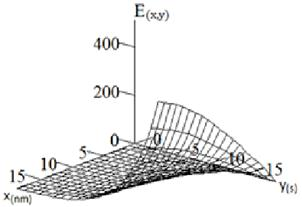Fig. 8. Wave function ψE(x,y) as a function of position and time when b = 0.2 for imaginary part for b=0.2.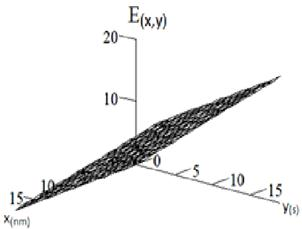Fig. 9. Wave function ψE(x,y) as a function of position and time for real part when b = 0.002.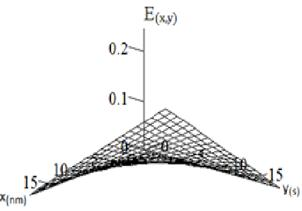Fig. 10. Wave function ψE(x,y) as a function of position and time when b = 0.002 for imaginary part for b=0.002.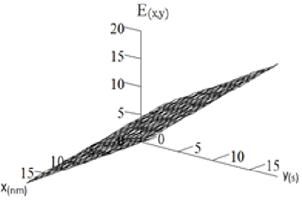Fig. 11. Wave function ψE(x,y) as a function of position and time for real part when b = 10-13.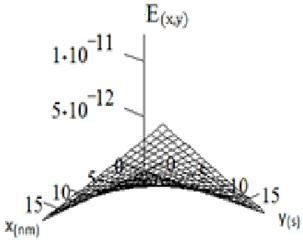Fig. 12. Wave function ψE(x,y) as a function of position and time when b =10-13 for imaginary part.

3. Result/Discussion

The expression for polarizability, b in terms of the electronic orientation was obtained from the Poisson’s equation that was derived from Maxwell’s equation. This formed the main basis of where the analysis is based on. The imposition of the polarizability terms on the propagating wave culminated from the fact that the solution of the Poison’s equation enabled us to obtain the constant which was the ingredient in the analysis. The real and complex wave function obtained were analyzed based on the effect of the polarizability on the propagated wave through the material. From the analysis, it was observed from fig. 1 and fig. 2, that the field profile was fractal in for both real and complex part of the result as obtained in equations (25) when the polarizability is unity. However, the fractal shape of the profile as shown in figs. 3 and 4 changed pattern as the value of the polarizability, b was increased to 2. The same trend went on when b was increased to 5. There was an observed change on the profile when b was remarkably decreased to 0.2, milimetre value and subsequently to micron value (0-002). Here the profile made an inclination to with the y- component as b was further decreased to 0.002 as in figs. 7 and 9. However, when b was further decreased to tetra (10-12) value, the inclination remained stabilized which is an indication that the threshold value of the polarizability had been attained. This also depicts the fact that the polarizability is not a linear function as it depends on so many factors including temperature the dielectric nature of the material.

4. Conclusion

From the study, we successfully derived Poisson’s equation that contains dielectric constant with an element of polarizability terms from combination of 1 and 3 of Maxwell equations that was solved to obtain the expressions that depicted effect of the polarizability on both real and complex parts of electromagnetic field propagated through oxide based thin film with reference to copper oxide thin film. In the analysis, the influence of the polarizability on the profile was made manifest as depicted on the graphs where the behavour of the graphs changed with the variation of the polarizability terms though not in linear manner.

References

1. Ekaterina I., Izgorodina, Maria Forsythb and Douglas R. MacFarlane, Phys. Chem-Chem. Phy 2009, 11 2452-2458
2. E. I Ugwu and H. M Ogbu PJST, 2008, 9 (1) 189–195
3. D. C Dhukarya, Proceedings of the International Mulnconference of Engineering and Computer Scientists, III IMECS Vol. 2010
4. H. Weingartner, P. Sasisanker, C. Daguenet, P. J. Dyson, I. Krossing, J. M. Slattery and T. Schubert, J. Phys. Chem. B, 2007, 111, 4775–4780.
5. S. O. Pillai, Solid State Physics, New International (P) Physics Publishers, Sixth Ed. 2010, 635
6. C. Wakai, A. Oleinikova, M. Ott and H. Weingartner, J. Phys. Chem. B, 2005, 109, 17028–17030.
7. H. Weingartner Z. Phys. Chem., 2006, 220, 1395–1405.
8. S. Schrodle, G. Annat, D. R. MacFarlane, M. Forsyth, R. Buchner and G. Hefter, Chem. Commun, 2006, 1748–1750.
9. S. Schrodle, G. Annat, D. R. MacFarlane, M. Forsyth, R. Buchner and G. Hefter, Aust. J. Chem., 2007, 60, 6–8.
10. A. Stoppa, J. Hunger, R. Buchner, G. Hefter, A. Thomas and H. Helm, J. Phys. Chem. B, 2008, 112, 4854–4858.

 Contents 1. 2. 3. 4.
Article ToolsAbstractPDF(1021K)